# How to Calculate and Solve for Net Heat Flux for Radiation | Radiation Heat Transfer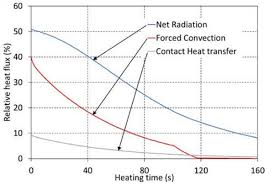The image above represents net heat flux for radiation.

To compute for net heat flux for radiation, four essential parameters are needed and these parameters are Emissivity (ε), Area (A), Emitting Power of Black Body (eb) and Radiosity (J).

The formula for calculating net heat flux for radiation:

qnet = εAeb / 1 – ε – J

Where:

qnet = Net Heat Flux for Radiation
ε = Emissivity
A = Area
eb = Emitting Power of Black Body

Let’s solve an example;
Find the net heat flux for radiation when the emissivity is 22, the area is 2, the emitting power of black body is 10 and the radiosity is 14.

This implies that;

ε = Emissivity = 22
A = Area = 2
eb = Emitting Power of Black Body = 10

qnet = εAeb / 1 – ε – J
qnet = (22)(2)(10) / 1 – 22 – 14
qnet = 440 / -21 – 14
qnet = -20.9523 – 14
qnet = -34.95

Therefore, the net heat flux for radiation is -34.95 W.

Nickzom Calculator – The Calculator Encyclopedia is capable of calculating the net heat flux for radiation.

To get the answer and workings of the net heat flux for radiation using the Nickzom Calculator – The Calculator Encyclopedia. First, you need to obtain the app.

You can get this app via any of these means:

To get access to the professional version via web, you need to register and subscribe for NGN 2,000 per annum to have utter access to all functionalities.
You can also try the demo version via https://www.nickzom.org/calculator

Apple (Paid) – https://itunes.apple.com/us/app/nickzom-calculator/id1331162702?mt=8
Once, you have obtained the calculator encyclopedia app, proceed to the Calculator Map, then click on Materials and Metallurgical under Engineering.Now, Click on Radiation Heat Transfer under Materials and MetallurgicalNow, Click on Net Heat Flux for Radiation under Radiation Heat Transfer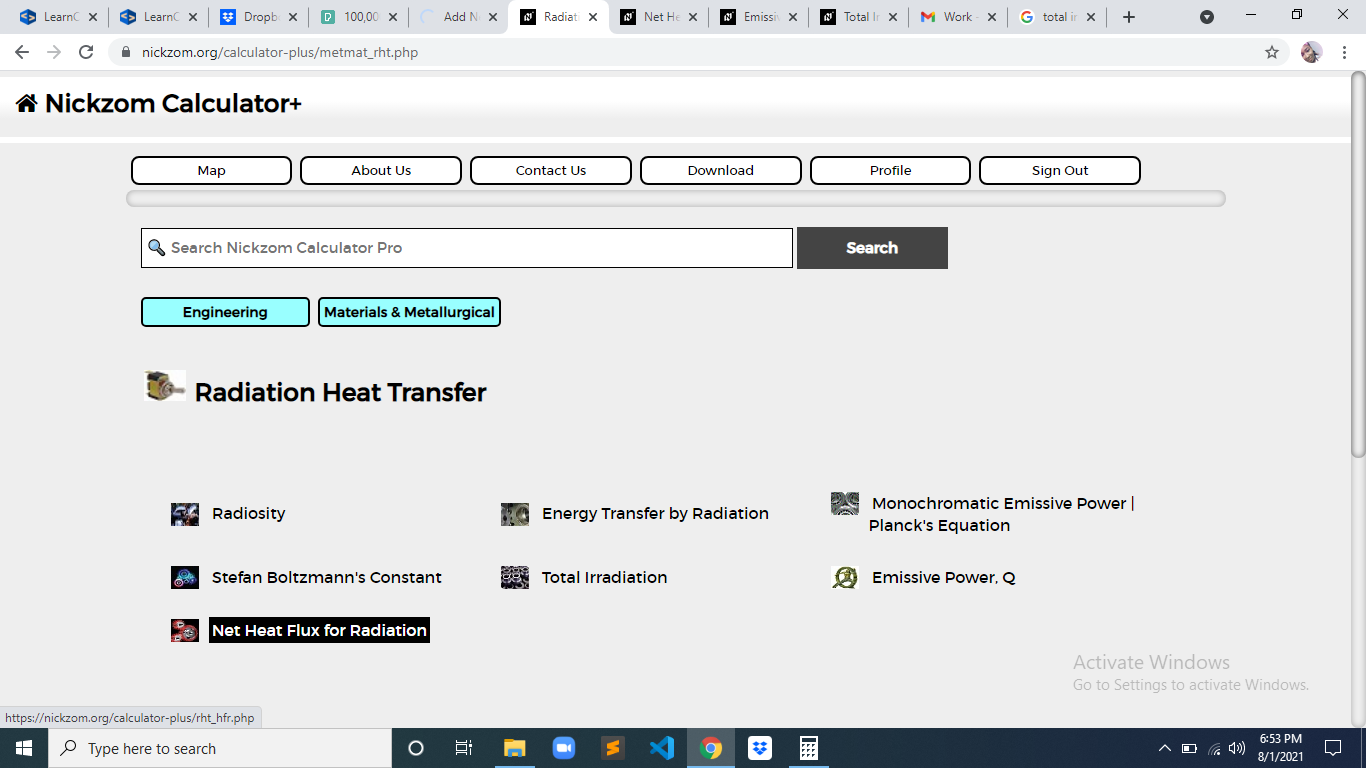The screenshot below displays the page or activity to enter your values, to get the answer for the net heat flux for radiation according to the respective parameter which is the Emissivity (ε), Area (A), Emitting Power of Black Body (eb) and Radiosity (J).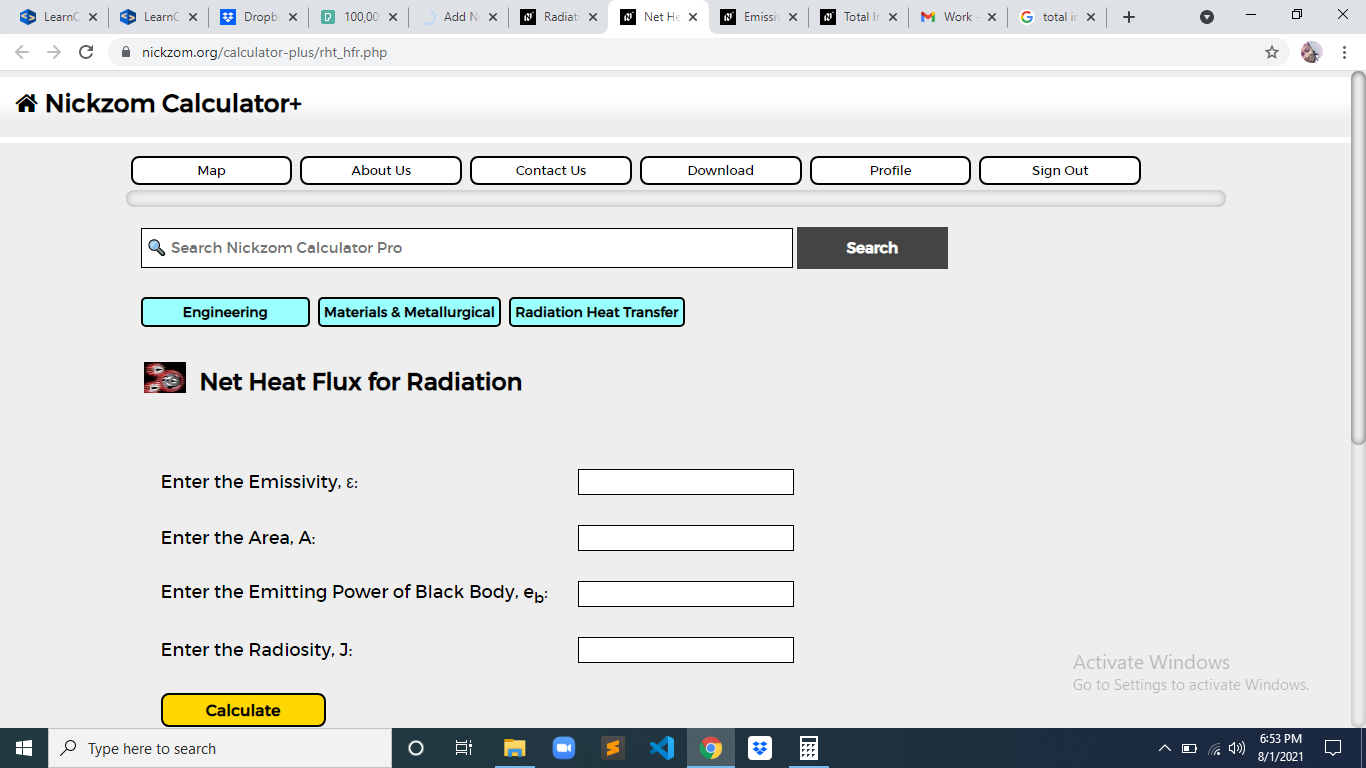Now, enter the values appropriately and accordingly for the parameters as required by the Emissivity (ε) is 22, Area (A) is 2, Emitting Power of Black Body (eb) is 10 and Radiosity (J) is 14.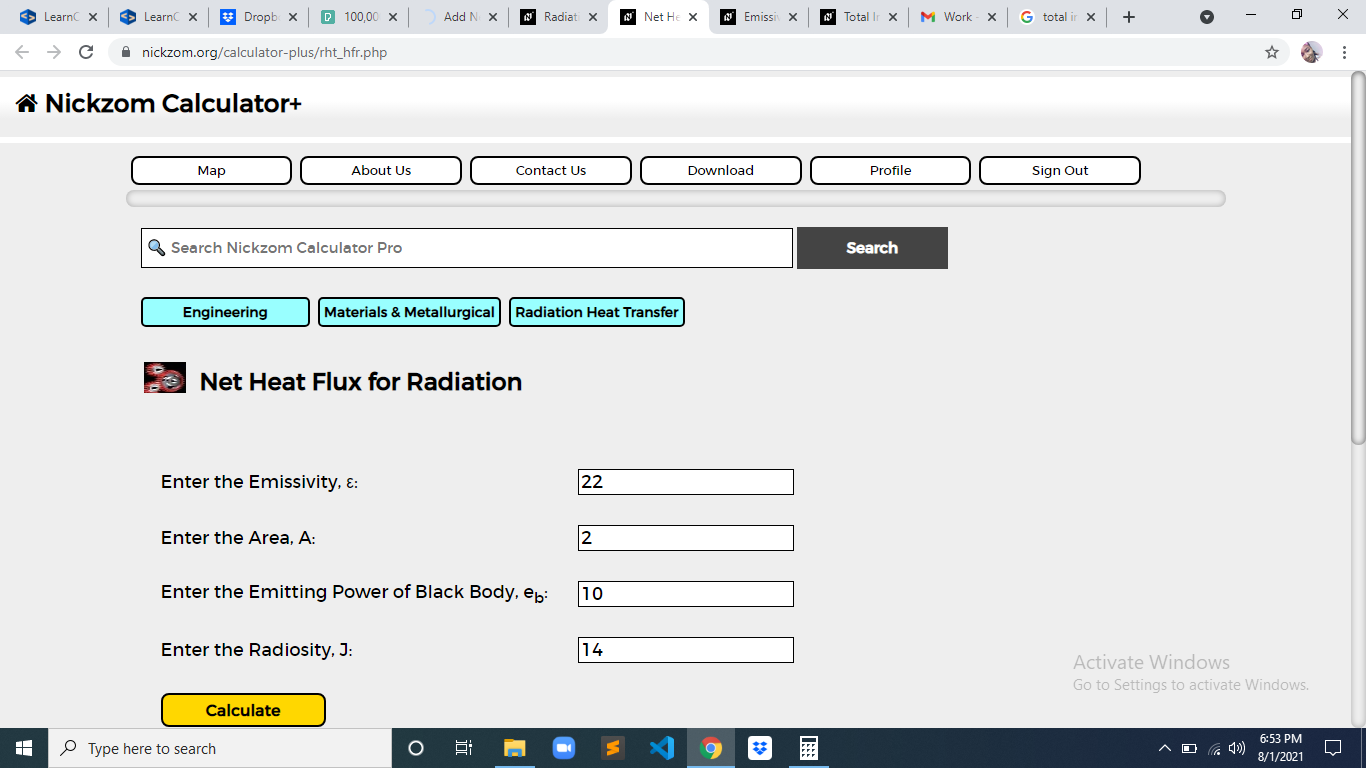Finally, Click on Calculate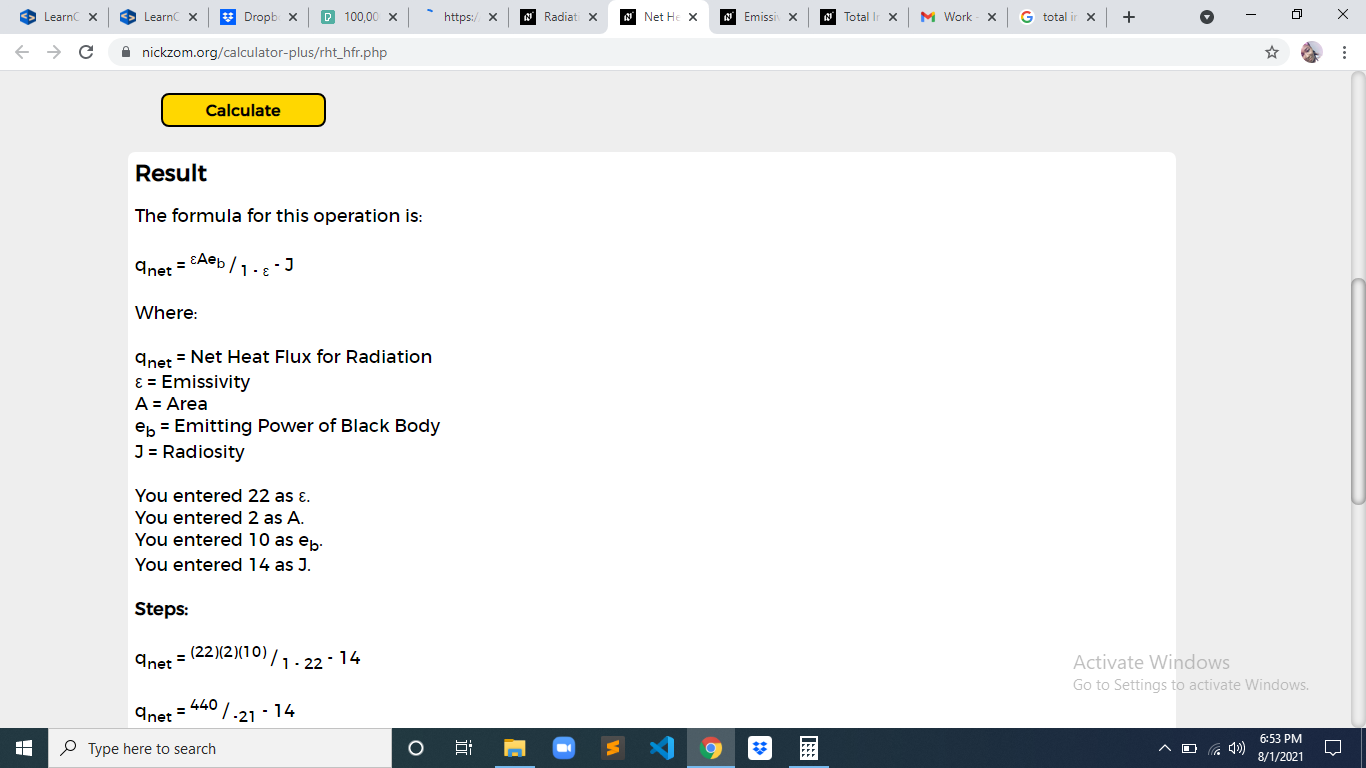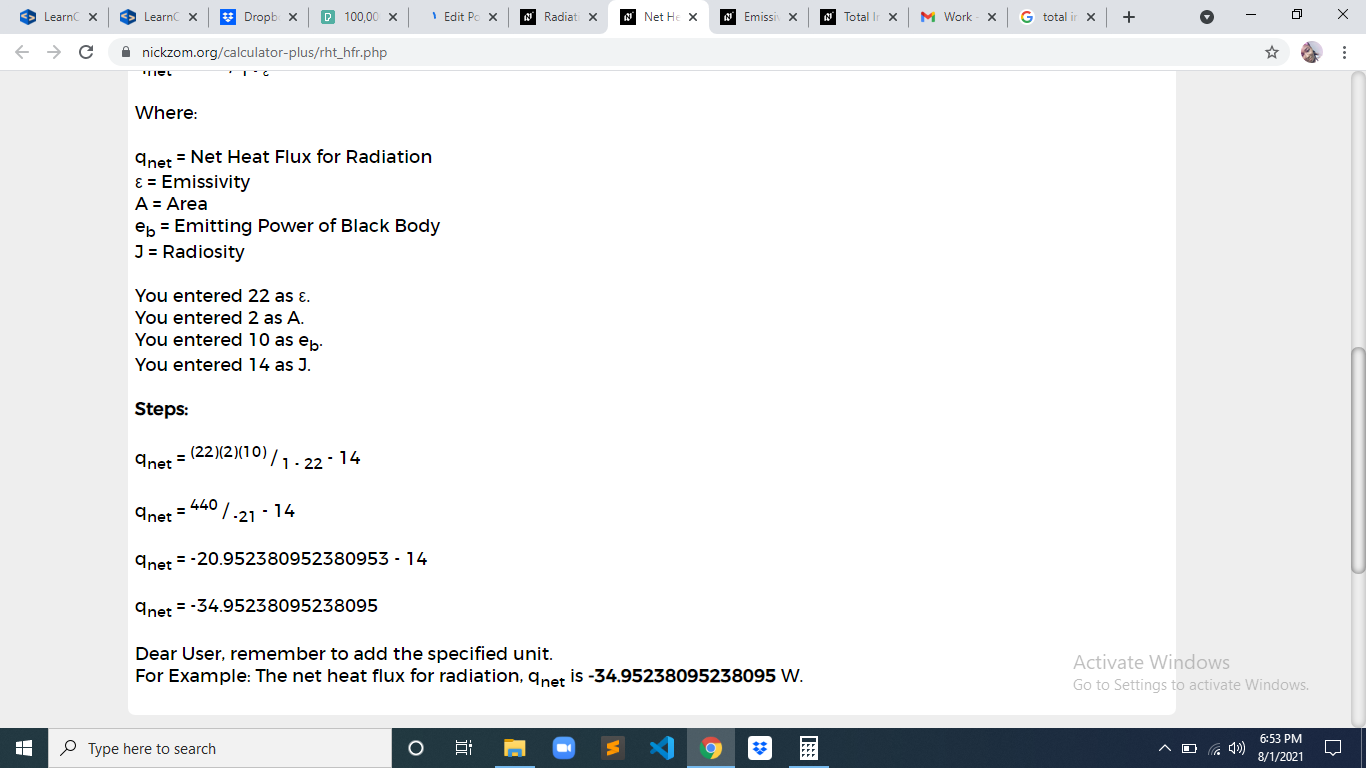As you can see from the screenshot above, Nickzom Calculator– The Calculator Encyclopedia solves for the net heat flux for radiation and presents the formula, workings and steps too.